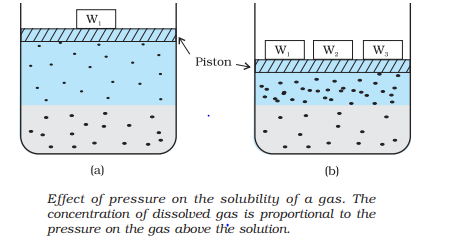# solubility

## Solubility

• It is the maximum amount that can be dissolved in a specified amount of solvent at a specified temperature.
• It depends upon the nature of solute and solvent as well as temperature and pressure.

### Solubility of a Solid in a Liquid

Saturated solution
It is a solution in which no more solute can be dissolved at the same temperature and pressure.
Unsaturated Solution
An unsaturated solution is one in which more solute can be dissolved at the same temperature
How is solubility determined
The solution which is in dynamic equilibrium with undissolved solute is the saturated solution and contains the maximum amount of solute dissolved in a given amount of solvent. The concentration of solute in such a solution is its solubility
Effect of Temperature
• In a nearly saturated solution if dissolution process is an endothermic process, solubility increases with increase in temperature.
• In a nearly saturated solution if dissolution process is an exothermic process, solubility decreases with increase in temperature.

### Solubility of a Gas in a Liquid

Solubility of gases in liquids is greatly affected by pressure and temperature.
Effect of Pressure
The solubility of gases increase with increase of pressure.Henry’s Law
It states is two ways
“ the solubility of gas in a liquid is directly proportional to the partial pressure of the gas present above the surface of liquid or solution”.,
or
“the partial pressure of gas in Vapour phase is proportional to the mole fraction of the gas in the solution”.
$p = K_H \chi$
Here $K_H$ is the Henry’s law constant
Effect of Temperature
Solubility of gases in liquids decreases with rise in temperature

## Solved examples

Example 1
If $N_2$ gas is bubbled through water at 293 K, how many millimoles of gas would dissolve in 1 litre of water? Assume that gas exerts a partial pressure of 0.987 bar. Given that Henry’s law constant for gas at 293 K is 76.48 kbar.
Solution
The mole fraction of the gas in the solution is calculated by applying Henry’s law.
$p = K_H \chi$
$\text{Mole Fraction of Nitrogen} = \frac {\text{partial pressure of nitrogen}}{K_H = 0.987/76480= 1.29 \times 10^{–5}$
As 1 litre of water contains 55.5 mol of it, therefore if n represents number of moles of $N_2$ in solution,
$1.29 \times 10^{–5} = \frac {n}{{n+ 55.5}$
(n in denominator is neglected as it is < < 55.5)
Thus $n = 1.29 \times 10^{–5} \times 55.5=7.16 \times 10^{–4}$ mol